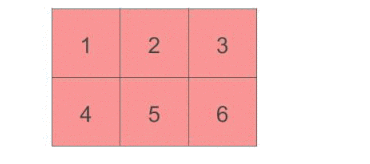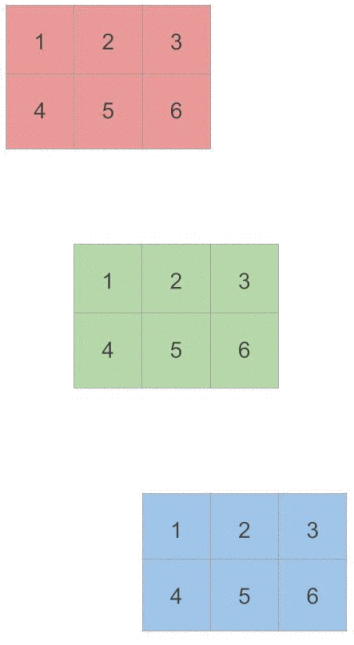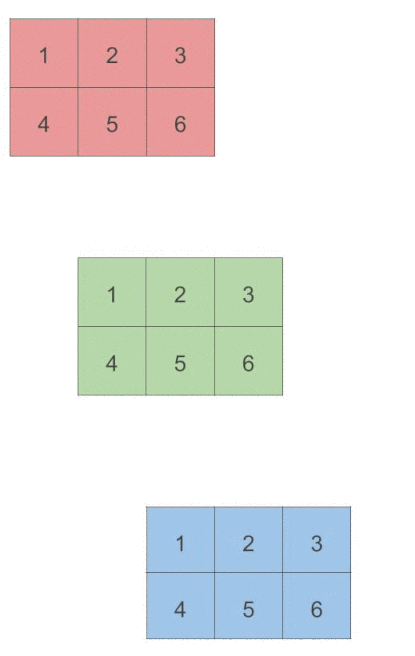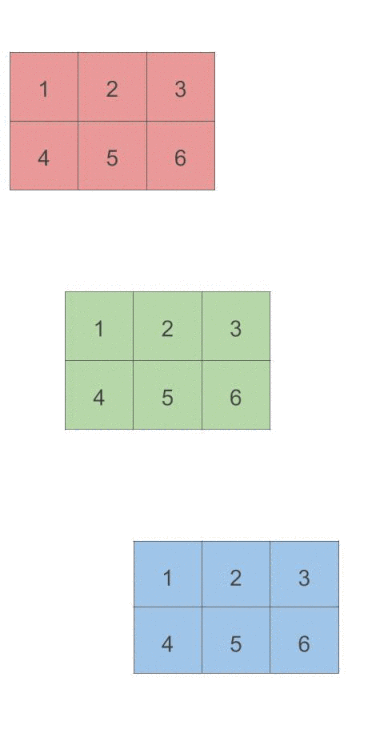# 理解 PyTorch 中维度的概念2020年4月11日07:51:51

32 2933字阅读9分46秒

## 理解 PyTorch 维度概念

1. x = torch.tensor([
2.         [1,2,3],
3.         [4,5,6]
4.     ])
5. # 我们可以看到"行"是dim=0, "列"是dim=1
6. print(x.shape)
7. >> torch.Size([2, 3])

• first dimension (dim=0) stays for rows, 第一个维度代表行, 因为是2, 实际x就是2行
• the second one (dim=1) for columns, 第二个维度代表列, 因为是3

1. torch.sum(x, dim=0)
2. >> tensor([5, 7, 9])

1. torch.sum(x, dim=1)
2. >> tensor([ 6, 15])

The way to understand the "axis" of numpy sum is that it collapses the specified axis. So when it collapses the axis 0 (the row), it becomes just one row (it sums column-wise).### 对于三维向量

1. # 看一下三维的
2. x = torch.tensor([
3.         [
4.          [1,2,3],
5.          [4,5,6]
6.         ],
7.         [
8.          [1,2,3],
9.          [4,5,6]
10.         ],
11.         [
12.          [1,2,3],
13.          [4,5,6]
14.         ]
15.     ])
16. # 我们可以看到第三维是dim=0, "行"是dim=1, 列是dim=2
17. print(x.shape)
18. >> torch.Size([3, 2, 3])

1. torch.sum(x, dim=0)
2. >>
3. tensor([[ 3,  6,  9],
4.         [12, 15, 18]])1. torch.sum(x, dim=1)
2. >>
3. tensor([[5, 7, 9],
4.         [5, 7, 9],
5.         [5, 7, 9]])1. torch.sum(x, dim=2)
2. >>
3. tensor([[ 6, 15],
4.         [ 6, 15],
5.         [ 6, 15]])## 关于TopK的问题

1. # 看一下二维的
2. x = torch.tensor([
3.     [0.1, 0.2 ,0.5, 0.2],
4.     [0.4, 0.3, 0.2, 0.1],
5.     [0.1, 0.2, 0.5, 0.1],
6. ])
7. # -------
8. print(x.shape)
9. >> torch.Size([3, 4])

1. a, b = x.topk(1, dim=1)
2. print(a)
3. print('-'*10)
4. print(b)
5. print(b.shape)
6. >>
7. tensor([[0.5000],
8.         [0.4000],
9.         [0.5000]])
10. ----------
11. tensor([,
12.         ,
13.         ])
14. torch.Size([3, 1])

1. a, b = x.topk(1, dim=0)
2. print(a)
3. print('-'*10)
4. print(b)
5. print(b.shape)
6. >>
7. tensor([[0.4000, 0.3000, 0.5000, 0.2000]])
8. ----------
9. tensor([[1, 1, 0, 0]])
10. torch.Size([1, 4])

• 微信公众号
• 关注微信公众号
•• QQ群
• 我们的QQ群号
•• 本文由 发表于 2020年4月11日07:51:51
• 转载请务必保留本文链接：https://mathpretty.com/12065.html